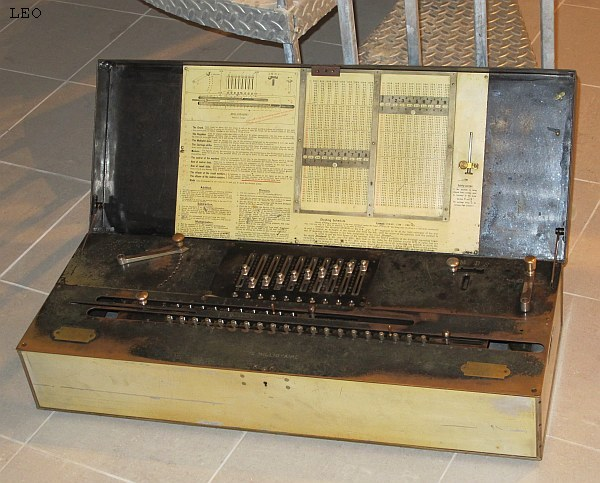previous <<==>> next

THE  MILLIONAIRE  CALCULATOR
WITH  INSTANT  MULTIPLICATION*  See the DEMONSTRATION by CLIFF STOLL on YouTube ...

See inside its beautiful brass machinery:
Have a look at the Restoration Procedures ...
 ``` T E C H N I C A L D A T A of the Mechanical Calculator » THE MILLIONAIRE « ******************************************************************************** Serial-Number: 2607 Dimensions: (ca.) Width = 31" / 79 cm Depth = 11_1/2" / 29 cm Height = 7 " / 18 cm Weight: (ca.) 82_1/2 lbs / 37.5 kg Mechanics: Instant-Multiplication Module / "1x1-KOERPER" Functions: Add, Subtract, Multiply, Divide Registers: Input = 10 Decimals Counter = 10 Decimals ( NO Carry ) Arithmetic = 20 Decimals Manufacturer: HANS W. EGLI Fabrikation von Rechenmaschinen Patent O. Steiger Zurich / Switzerland 1900 BASICS & HOW TO USE » THE MILLIONAIRE « ****************************************** Make sure, that the upper-right CRANK is in its HOME POSITION Pull the HANDLE and swing the REGULATOR to: A = Addition, M = Multiplication, D = Division, S = Subtraction For Addition & Subtraction set the MULTIPLIER to "1" PRESS the HANDLE of the CARRIAGE and SHIFT IT to the MIDDLE - than Pull it CLEAR COUNTER- & ARITHMETIC-Units by pulling their LEVERS to the RIGHT ADDITION & SUBTRACTION: ======================= Example: 123 + 45 - 6 = 162 CLEAR input, counter and arithmetic units; Set MULTIPLIER = "1" ADD = Set REGULATOR to "A" : Enter the first number (123) in the far right of the input unit. Make a (clockwise) turn with the crank to transfer the number into arithmetic unit. The counting unit displays the figure 1. Enter the second number (45). Make a turn with the crank to add the number. The arithmetic unit displays the intermediate sum (168) and the counting unit displays the figure 2. SUBTRACT = Set REGULATOR to "S" : Enter the third number (6). Make a turn with the crank. The arithmetic unit displays the result (162) and the counting unit is decreased by 1. REMARK: NEGATIVE RESULTS are displayed in the arithmetic unit as the COMPLEMENT of the next higher 10, 100, 1000, ... Example: -12 = 99...9988 MULTIPLICATION: =============== Example: 123 x 45 = 5535 CLEAR input, counter and arithmetic units; SHIFT the CARRIAGE to the RIGHT; Set REGULATOR to "M" : Enter the multiplicand (123) in the far right of the input unit. The (45) multiplicator has two digits. Start with the left-most figure (4), set the MULTIPLIER (= "4"), make O N E T U R N with the crank. Set the MULTIPLIER (= "5") to the next-right figure of the multiplicator (5), make O N E T U R N with the crank. THE M U L T I P L I C A T I O N I S D O N E !!! The multiplicand (123) stays in the input unit, the multiplicator (45) in the counter and the result (5535) is in the arithmetic unit. DIVISION: ========= Example: 22 : 7 = 3.1428571... SORRY, BUT ... The 2 PROCEDURES described in the MILLIONAIR's LID are strange. Suggestion: Apply the " N O R M A L P R O C E D U R E " ... CLEAR input, counter and arithmetic units; SHIFT the CARRIAGE to the RIGHT; Set MULTIPLIER = "1" ... and continue in the way of the T.I.M. ... Have a look at "Calculating Trickies" ... R E M A R K S : The brass labels left & right say: +------------------------+ +-------------------------------------+ | W. A. Morschhauser | | Hans W. Egli | | SOLE AGENT | | Fabrikation von Rechenmaschinen | | 1 Madison Avenue | | Pat. O.Steiger | | NEW YORK CITY | | ZUERICH | +------------------------+ +-------------------------------------+ Inside the LID are INSTRUCTIONS & MULTIPLICATION TABLES, used for "Division". There are 2 SCREWS stored to LOCK CARRIAGE & MULTIPLICATION-Module for when the calculator is shipped. This »MILLIONAIRE« has a METAL case. Especially the bottom plate is chipping like slate: After 100 years the metal is detoriating. Have a look at the Restoration Procedures ... impressum: ******************************************************************************** © C.HAMANN http://public.BHT-Berlin.de/hamann * 11/23/10 ```